## Forum Posts

Dimensional consciousness(LC)
LC
Aug 29, 2021
In Discussion
This is the phi yantra, discovered by Knew Geometry, I found it very interesting and overlayed the 10 tree grid kathara/fractaling square which I am currently researching.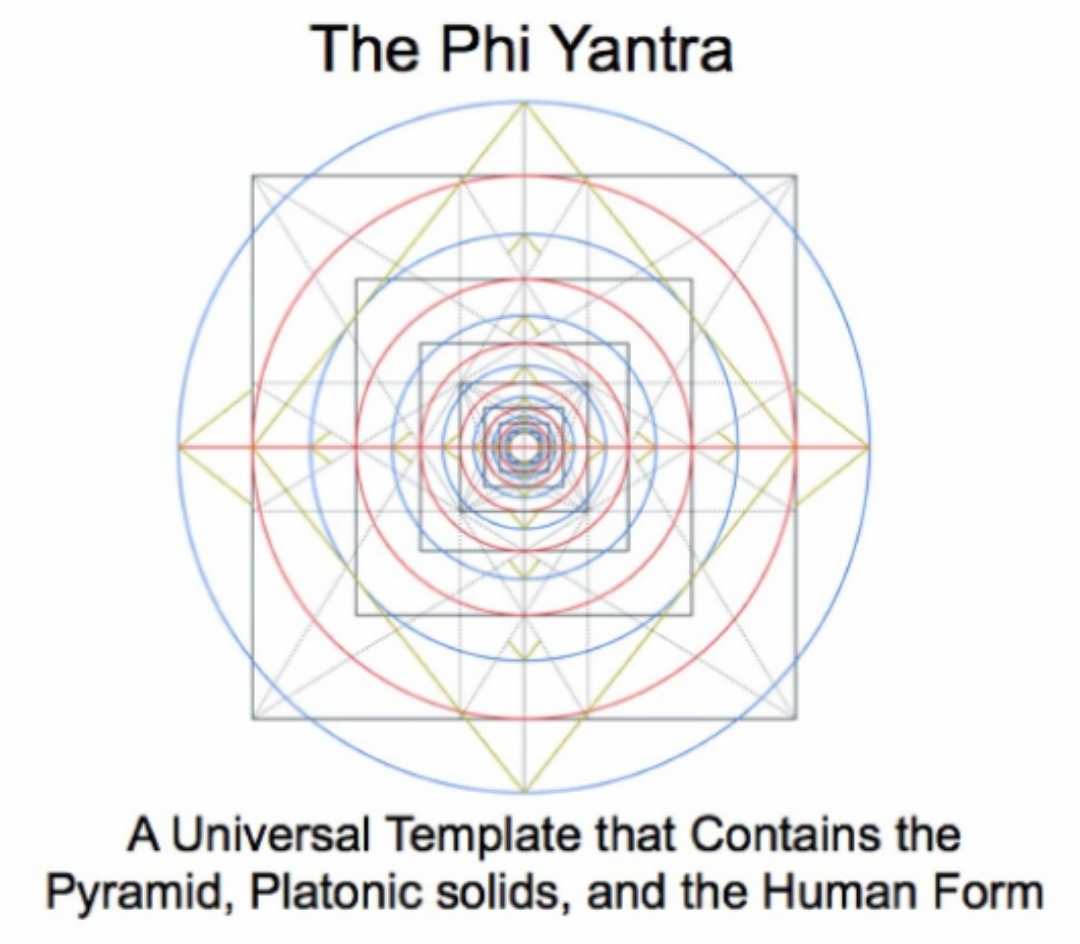0
0
10
Dimensional consciousness(LC)
LC
Aug 29, 2021
In Discussion
The fractaling square forms/produces the 12 tree grid but it only produces the first 10 points so you have to double the fractaling square or quadrupled the 12 tree grid to get the 2 and 11 points to form 12 points so it actually produces the 10 sephirot tree of life, this is because a structure needs to be formed to build the 12 vibrational dimensions on, this structure being the 10 spacetime dimensions which can be seen in the Pascals triangle tetractys which is the 10 points/spacetime dimensions which encodes a hexagon with a value of 12 which is the 12 vibrational dimensions and this hexagon is the seed of life which has two 3D forms which are the cubistic matrix which is formed out of the 12 tree grid and the vector equilibrium(VE)/cube octahedron which is the 3D doubled fractaling square formed out of the 12 tree grid and the VE is 12 spheres surrounding 1 meaning the hexagon, which forms the 12, and the 12 tree grid are 12 surrounding 1 so the tree of life is 13 sephirot which can therefore be represented in a hexagonal form AKA the hexatree which encodes the 12 tree grid in 3D (when it rotates). The hexatree encodes the fractaling star tetrahedron which grows by doubling(Binary) but encodes the Fibonacci spiral(Trinary) which explains why the spiral forms a binary-trinary sequence, "each level of the hypercube fractal corresponds to a hexagon(hexagonal number)/cube in the flower of life(tetrahedron grid(CO/VE) fractal)/cubistic matrix which is formed out of the 12 tree grid, these (hyper)cube-hexagon/flower of life levels are the cubes in the cube octahedron(CO/VE) fractal which is/are formed/produced out of and form the Fibonacci spiral, the CO/VE/cubistic matrix encodes/produces both the Fibonacci and Krystal spirals". "As we already know there is a connection between the 13 sephirot(dimensions), 15 dimensions(12 tree grid) and 16 sephirot(dimensions) within the torus so there is obviously a connection between the 12 tree grid and 13 sephirot hexatree, the 12 tree grid forms the Krystal spiral which is binary whilst the 13 sephirot hexatree forms the Fibonacci spiral which is trinary, this shows the binary-trinary connection which can be seen in 2D/3D geometry because the 12 tree grid-13 sephirot hexatree=2D star tetrahedron(grows by binary)-3D star tetrahedron(grows by trinary) so the 13 sephirot hexatree is the 12 tree grid in 3D(?) which makes sense because the 12 points surround the 1 which is the 12 tree grid sephirot around the 13th sephirot, "The seed (of life), 6 as 1, 6 surrounding 1, the 7 sephirot of creation, when it expands forms 12 circles corresponding to the 12 tones in an octave and the tetractys, the hexagonal yod of the pascals triangle tetractys is equal to 12, the hexagonal yod is the 7/seed of life... the 12 circles surrounding the seed AKA 1, 12 as 1, the center of the seed..."... The hexatree structure being a hexagon within a hexagon can be seen as a cube within a cube which is a hypercube, showing the 13 sephirot hexatree and 12 tree grid connection, and since the hexatree is a hypercube that means it is the PNC-hypercube and can therefore be formed out of the 4-hexatree which links into the 5:6 correspondence because the hexatree is the 6 in the 5:6 correspondence(which is the 10(tetractys) dimensions:12 dimensions correspondence) and the 5 corresponds to pell numbers/octagram-PNC-hypercube square." Picture: Tree of life encoded in hexatree(13 sephirot tree of life). Tree of life+1 yellow "sephirot"(Da'at)+green sephirot=12 tree grid encoded in hexatree. Tree of life+3 Yellow sephirot=13 sephirot tree of life encoded in hexatree. 13 sephirot tree of life+green and pink sephirot=15 tree grid encoded in hexatree.0
0
2
Dimensional consciousness(LC)
LC
Aug 29, 2021
In Discussion
Stephen M Phillips shows the overlapping circles, that form the (outer) tree of life, form the 7 polygons which, in his work, are the inner tree of life. The flower of life(overlapping circles) form polygons, so I merged two seeds of life to form a hexagonal form of the tree of life and these seeds of life form the 7 polygons.0
0
1
Dimensional consciousness(LC)
LC
Aug 09, 2021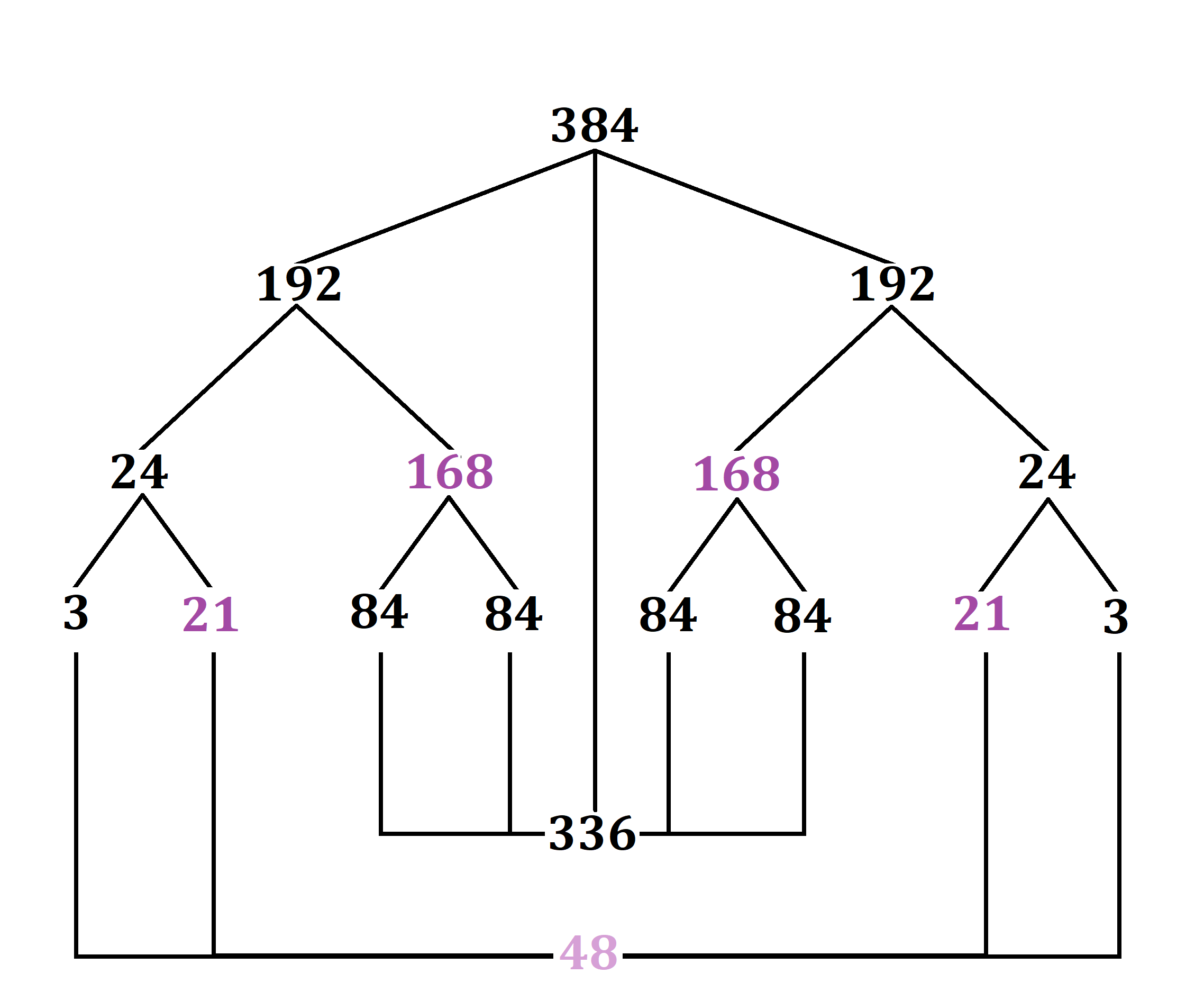0
0
2
Dimensional consciousness(LC)
LC
Aug 07, 2021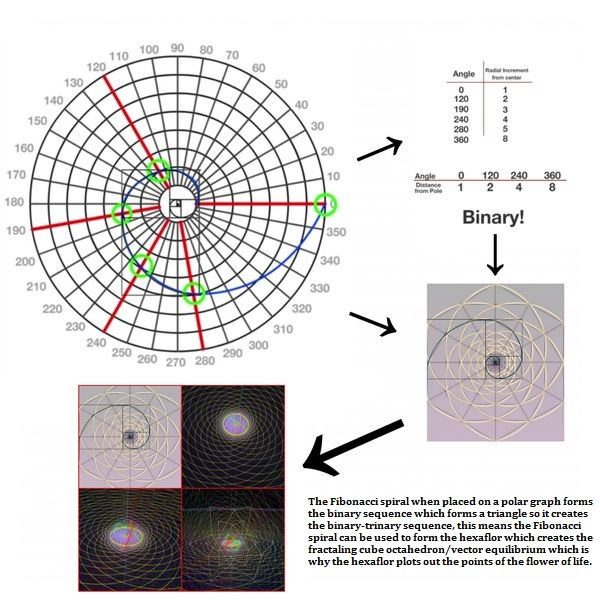0
0
4
Dimensional consciousness(LC)
LC
Aug 07, 2021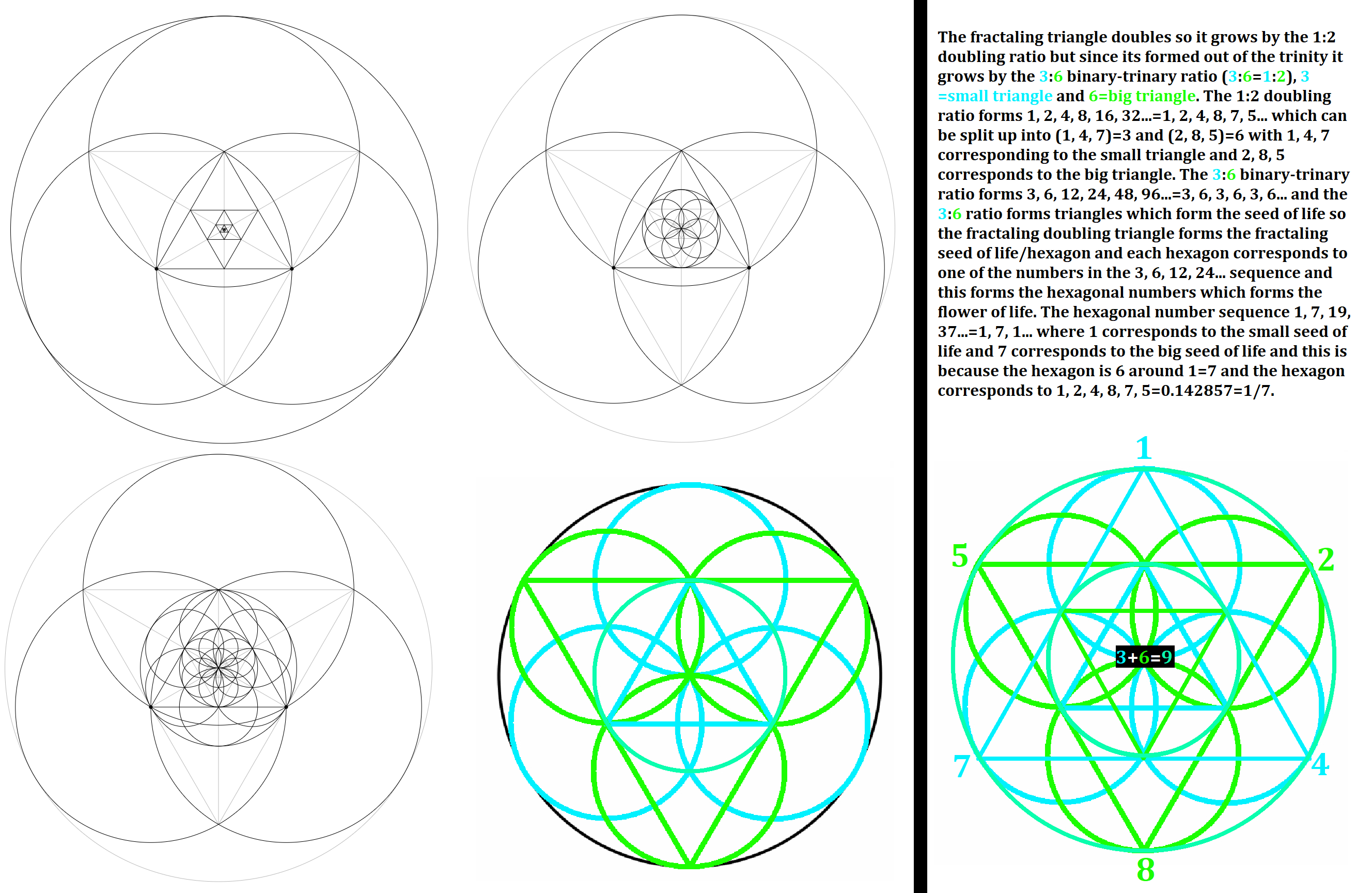0
0
1
Dimensional consciousness(LC)
LC
Jul 08, 2021
In Discussion
The debunking keylontic science diagram has been updated with the new information I have been posting for over a year, the diagram has also been redesigned:0
0
62
Dimensional consciousness(LC)
LC
Jun 11, 2021
In Discussion
Knew Spirituality showed the fractaling cube viewed as a hexagon produces the Fibonacci spiral, this is related to the cube octahedron producing the Fibonacci spiral so it is a Krystal-Fibonacci spiral. The fractaling cube/hexagon, which produces the Krystal-Fibonacci spiral, forms the hexagonal numbers and when we extend the hexagonal number sequence geometrically produces doubled fractaling hexagons.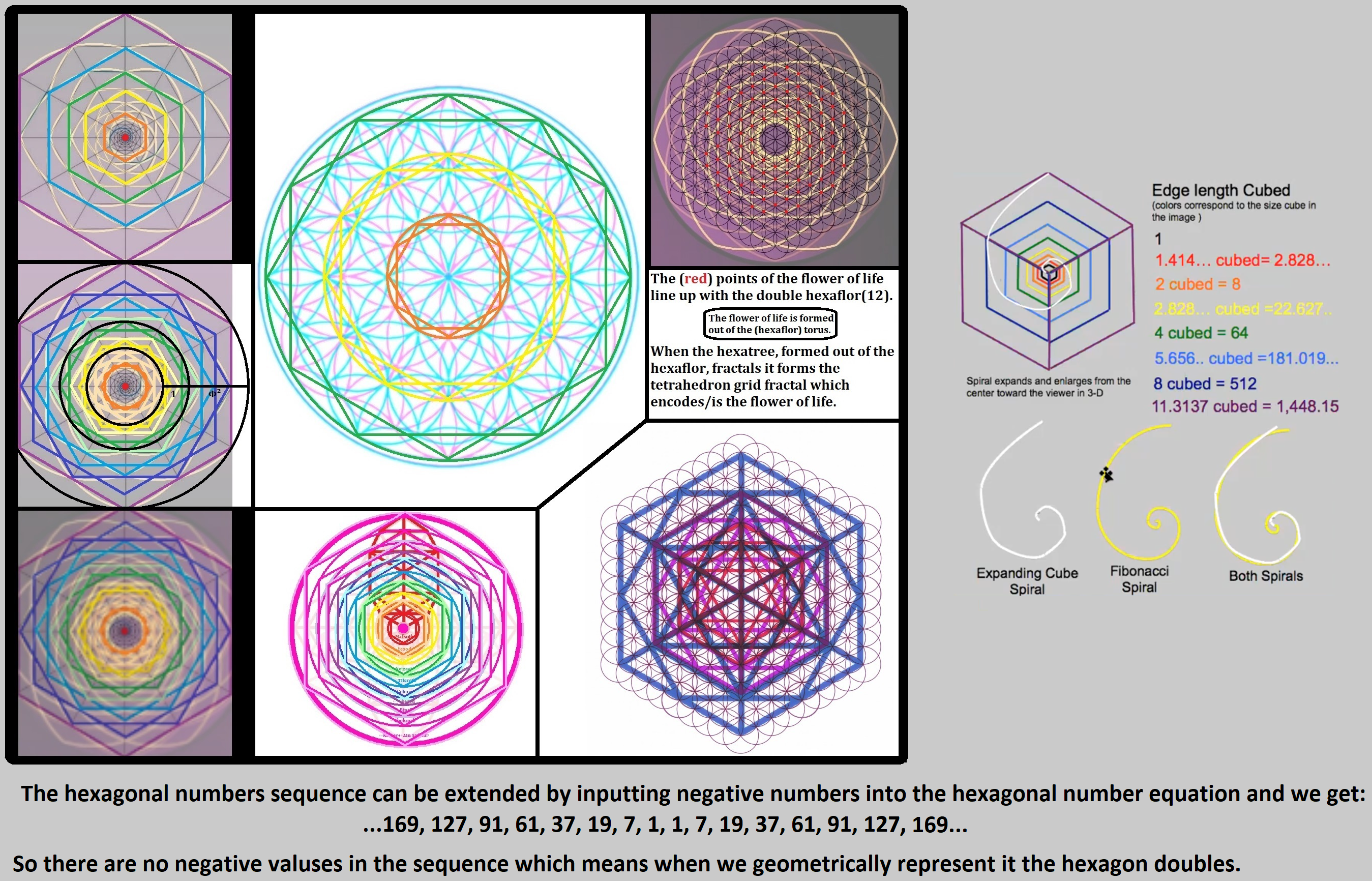0
0
7
Dimensional consciousness(LC)
LC
Jun 11, 2021
In Discussion
I have talked about the Krystal-Fibonacci spiral for a few years and I thought I was the only one to reveal it but finally I'm not! Knew Spirituality made a video 7 months ago revealing the exact same information I have been revealing and I want to thank them for that, its amazing! I created a new playlist on my personal youtube channel with there videos in it along with mine, I seriously suggest you check it out! The fractaling cube octahedron produces both the Fibonacci and Krystal spiral as I've shown. "The cube octahedron(vector equilibrium) fractal forms both the Fibonacci spiral (based torus) and the krystal spiral so it forms the krystal-fibonacci spiral. The hexatree forms the vector equilibrium, when it fractals it forms the Fibonacci spiral and spins and forms the torus (from the spinning flower of life formed out of the hexatree), this forms the cube octahedron fractal/PNC-hypercube." "FINALLY! I'm not the only one talking about this, I've been saying similar stuff for 2 years now but unfortunately some people have been attacking me for revealing this stuff. I've come up with the exact same information, I've recently made posts about it: Thank you for sharing this, its amazing! I would say there not exactly the same spiral but the cube octahedron fractal forms/encodes both the Fibonacci and Krystal spiral."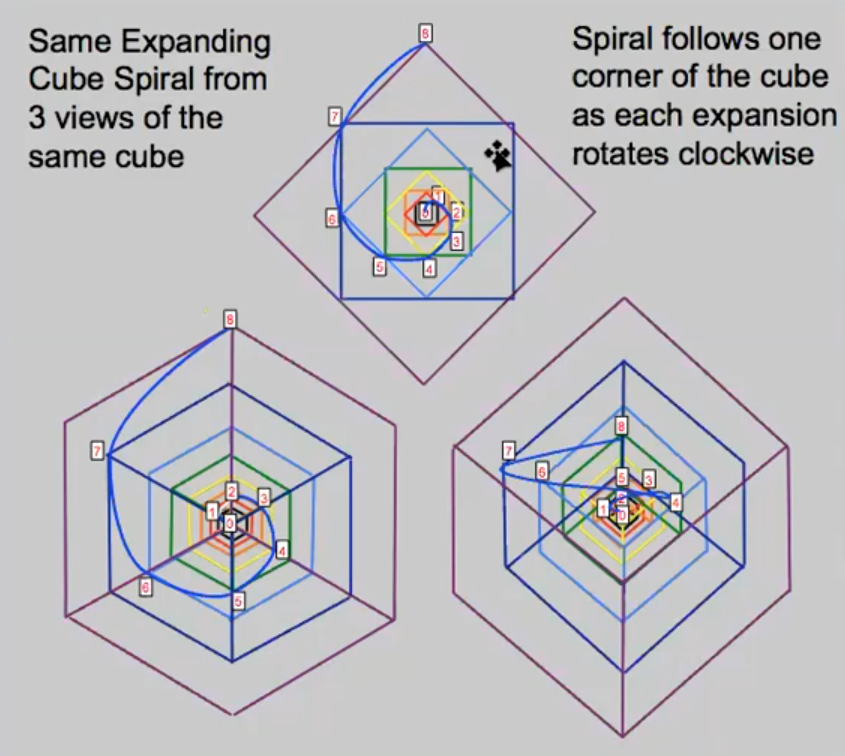0
0
48
Dimensional consciousness(LC)
LC
Jun 07, 2021
In Discussion
In a recent post I showed 117's connection to the silver ratio/pell numbers, 117 has some very interesting properties which I will be going over in a new diagram which was already in the making before I started creating the silver ratio diagram. The new diagram will be going over the numbers encoded in Ein Sof Aur, I've already gone over 1784 and 1577 in a previous diagram but wanted to look deeper into Ein Sof Aur anyway 117 is encoded in Ein Sof Aur in Ein in the form of 711 so I thought I would show you all some interesting properties of 117: The pell numbers repeating sequence can be formed out of the Fibonacci numbers that are divisible by 5 and the repeating sequence added up is 117. 117 is a pentagonal (5) number. 117 in base 5 is 432. If the area of a hexagon is 117 then the length of the radius and sides is 6.711, .711 being the revers/mirror of 117.
0
0
4
Dimensional consciousness(LC)
LC
Jun 03, 20210
0
4
Dimensional consciousness(LC)
LC
May 29, 2021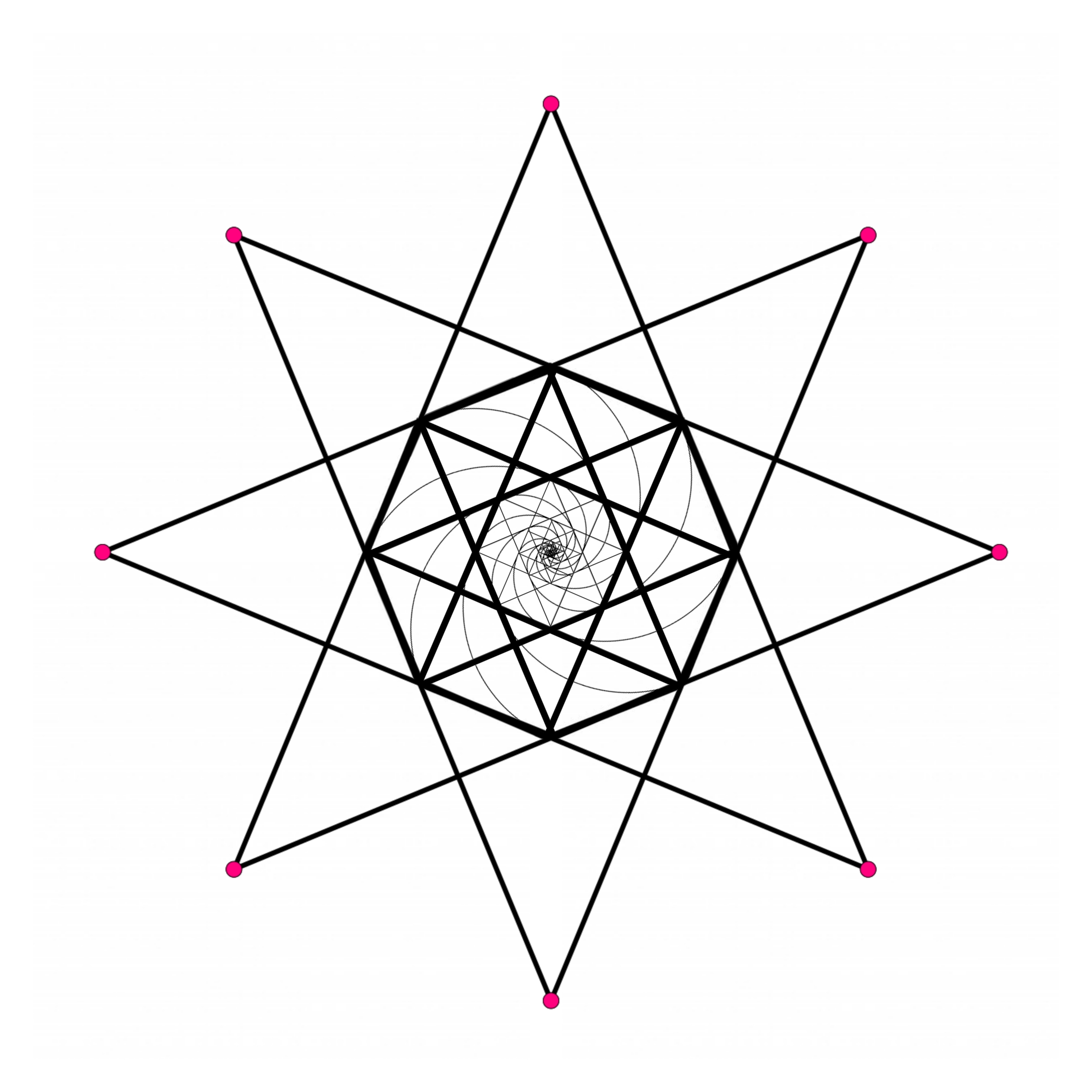0
0
4
Dimensional consciousness(LC)
LC
Apr 26, 20210
0
5
Dimensional consciousness(LC)
LC
Apr 13, 20210
0
10
Dimensional consciousness(LC)
LC
Apr 11, 2021
In Discussion
The torus is 12 circles surrounding 1, this forms 13 dimensions which corresponds to the hexatree, the hexatree is based off of the 3D kabbalistic tree of life which has 16 sephirot so we can place the trinity inside the 13th central circle therefore forming 16, the trinity forms a trinity on the outside adding 3 outer circle so 15 surrounded 1, yet another way of forming 16, this forms the 16 dimensional matrix. This means there are 16 dimensions.0
0
5
Dimensional consciousness(LC)
LC
Apr 10, 2021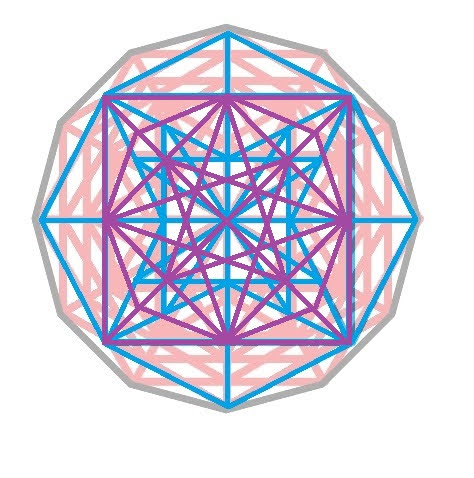0
0
19D